Some random parry method (Read 4384 times)

Started by Byakko, April 04, 2005, 04:28:56 pm

Byakko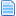Some random parry method
#1  April 04, 2005, 04:28:56 pm
••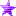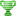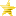Just thought I could drop this. This is originally based off some other methods I saw in other chars, then modified with few random fixes/changes...

[SP], [CP] and [AP] are the statedef number for the stand, crouch and air parry, [v] is a variable number you choose by yourself...

In the CMD file :
Code:
`;---------------------------------------------------------------------------; [PARRY]; Stand parry[State -1, stp]type = hitoverridetriggerall = statetype != A && command = "F"triggerall = movetype != Atrigger1 = ctrl || stateno = 850 || stateno = 851 || anim = 501 || stateno=[150,153]trigger1 = var([v]) := (1 + 3*(stateno = [150,153])); the +3 is to point out the red parry. Just eye-candy here, to glow red instead of blue.attr = SA, AA, APstateno = 850time = ifelse((stateno=[150,153]), 6, 8) ; ... for red parry, slightly shorter. Just because.slot = 1; Stand parry a crouching, but high, attack[State -1, stp]type = hitoverridetriggerall = statetype != A && command = "F"triggerall = movetype != Atrigger1 = ctrl || stateno = 850 || stateno = 851 || anim = 501 || stateno=[150,153]trigger1 = var([v]) := (1 + 3*(stateno = [150,153]))attr = C, AA, APstateno = 5005time = ifelse((stateno=[150,153]), 6, 8)slot = 2; Crouch parry[State -1, crp]type = hitoverridetriggerall = (statetype = S && command = "D") || (statetype = C && command = "F") ; ... yeah, I allow pressing forward ;_while_ crouching already. Because. Comment ?triggerall = movetype != Atrigger1 = ctrl || stateno = 850 || stateno = 851 || anim = 501 || stateno = [150,153]trigger1 = var([v]) := (2 + 3*(stateno = [150,153]))attr = CS, AA, APstateno = 851time = ifelse((stateno = [150,153]), 6, 8)slot = 1; Crouch parry a standing light attack[State -1, crp]type = hitoverridetriggerall = (statetype = S && command = "D") || (statetype = C && command = "Fw")triggerall = movetype != Atrigger1 = ctrl || stateno = 850 || stateno = 851 || anim = 501 || stateno = [150,153]trigger1 = var([v]) := (2 + 3*(stateno = [150,153]))attr = S, AA, APstateno = 5005time = ifelse((stateno = [150,153]), 6, 8)slot = 2; Air parry[State -1, aip]type = hitoverridetriggerall = statetype = A && command = "F"triggerall = movetype != Atrigger1 = ctrl || stateno = 852 || stateno = [154,155]trigger1 = var([v]) := (3 + 3*(stateno = [154,155]))trigger2 = Vel Y > 0 && StateNo = [1050,1201]trigger2 = var([v]) := (3 + 3*(stateno = [154,155]))attr = SA, AA, APstateno = 852time = ifelse((stateno = [154,155]), 6, 8); no parry if can't parry[State -1, ps]type = hitoverride; player has changed state (attack, no control...)trigger1 = !ctrl && stateno != [850,852]trigger1 = anim != 501 && stateno != [150,155] ; player has changed state - a stand parry got activated and the char jumps, have to deactivate stand parry...trigger2 = statetype = A && var([v]) != 3 && var([v]) != 6trigger3 = statetype = C && var([v]) != 2 && var([v]) != 5trigger4 = statetype = S && (var([v]) = 3 || var([v]) = 6)trigger5 = stateno = [100,110]attr = SCAtime = 0slot = 1[State -1, ps]type = hitoverridetrigger1 = !ctrl && stateno != [850,852]trigger1 = anim != 501 && stateno != [150,155]trigger2 = statetype = A && var([v]) != 3 && var([v]) != 6trigger3 = statetype = C && var([v]) != 2 && var([v]) != 5trigger4 = statetype = S && (var([v]) = 3 || var([v]) = 6)trigger5 = stateno = [100,110]attr = SCAtime = 0slot = 2`

CNS :
Code:
`;---------------------------------------------------------------------------; HITG_SHAKE[Statedef 5005]type    = Smovetype= Hphysics = Nvelset = 0,0[State 5005, stand parry check] ; checks if I'm trying to stand parry an attack that has...type = VarSettrigger1 = Var([v]) = 1 || Var([v]) = 4trigger1 = GetHitVar(AnimType) >= 4 ; ...  that has animtype = up or diagup.; since gethitvar(type) doesn't work, I couldn't check for crouch attacks that hit *high*... (such as Kyō's crouch HP) Bah.var([v]) = 10[State 5005, crouch parry check] ; you can crouch parry a light standing attack.type = VarSettrigger1 = Var([v]) = 2 || Var([v]) = 5trigger1 = !GetHitVar(AnimType)var([v]) = 11[State 5005, Clipboard]type = DisplayToClipboardtrigger1 = timetext = "GHVAnimType: %d"params = GetHitVar(AnimType)ignorehitpause = 1[state 5005, parry] ; because it's a movetype = H, you know.type = LifeAddtrigger1 = Var([v]) >= 10value = GetHitVar(Damage)[State 5005, parry] ; the changestate to the parry state.type = ChangeStatetrigger1 = var([v]) >= 10value = 840 + Var([v]) ; <= that's because of the state numbers I chose for my parries. Adjust at will.[after this, the rest is a straight copy of statedef 5000. So that it will behave normallywhen it turns out after the double-check that you can't parry.]`
Anim 501 is the end of power charge.
Comments, bug reports, be my guest...
One problem with this is, player hits forward when he doesn't have ctrl, releases, gets control back less than 6 ticks later, and gets hit and can't parry, and I guess he should parry in this case...
... Bah...

Most likely not the best method, just one amongst others. Because I felt like.

edit March 7 2006, because.
If I struggled to the end of my determination, to the end of my way of life with my followers, if the result is ruin, then this ruin is inevitable. Grieve. Shed tears. But you cannot regret.
Last Edit: March 07, 2006, 10:32:16 pm by Byakko

MasterstyleRe: Some random parry method
#2  April 07, 2005, 11:10:20 pm
•• Master of the Style
very interesting i'll test it thanks byakko!

ByakkoRe: Some random parry method
#3  March 07, 2006, 10:07:12 pm
••Updated after testing random different methods and some discussion on #mugen. Now with hot slot action for some more in-depth checking.
Give feedback if possible, of courseedit - fixed some random minor stuffs. Updating changing state/variable numbers is a mess.
If I struggled to the end of my determination, to the end of my way of life with my followers, if the result is ruin, then this ruin is inevitable. Grieve. Shed tears. But you cannot regret.
Last Edit: March 07, 2006, 10:33:52 pm by Byakko

PotSRe: Some random parry method
#4  March 08, 2006, 02:45:53 am
•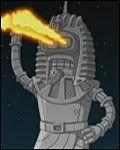•Use anything you want from my works.  If you need to contact me use email, not private messages.

ByakkoRe: Some random parry method
New #5  March 08, 2006, 08:47:44 am
••Quote
unless you want to be able to have more than one parry override active
Precisely.
Tap down, you will be able to parry both crouch attacks/projectiles, and stand light attacks (light attacks are checked in that custom state 5005). That's why I had to use 2 slots per parry (except air) to allow the second to go to the custom 5005 that checks the strenght of the attack through gethitvar(type). I wanted to allow to crouch parry stand low attacks, but gethitvar(animtype) doesn't work...

... Also, there's a way to make the parry closer to SF3 : the variable is used as a timer (let's say that between 10 and 0, it's a stand parry, between 20 and 10, crouch etc) so that you can disable the hitoverride (put another HOR on the same slot with time = 0) if you are still holding the direction at the 5th tick. SF3 gives a shorter parry time if you hold the direction.
If I struggled to the end of my determination, to the end of my way of life with my followers, if the result is ruin, then this ruin is inevitable. Grieve. Shed tears. But you cannot regret.
Last Edit: March 08, 2006, 08:49:24 am by Byakko